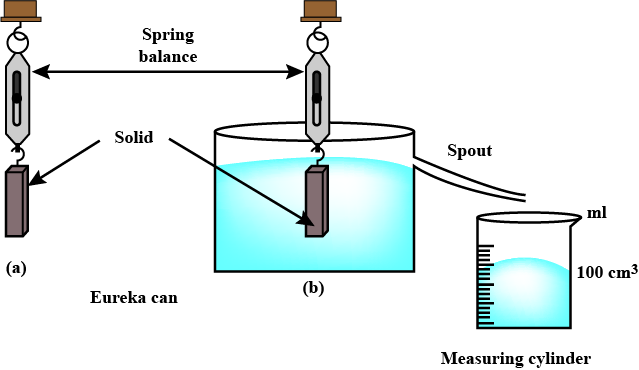# Archimedes’ Law Study Guide

INTRODUCTION

Fluids that surround an object exert forces on it that is known as the Archimedes’ principle. In the case of an object immersed in a fluid, its net weight is reduced in proportion to the applied force.

Greek Mathematician and Inventor Archimedes described it as an effect of immersion in a fluid (gas or liquid) at rest. He argued that any object immersed partially or entirely in a fluid would experience a buoyant force whose magnitude is equal to the amount of fluid displaced by it at rest.

## THE FORMULA FOR ARCHIMEDES’ PRINCIPLE

• The force exerted on an object equals the fluid’s weight misplaced by the object itself in its simplest form, according to Archimedes’ law.

Fb = ρ x g x V = ρgV

Where,

• Fb is the buoyant force,
• ρ is the fluid density,
• V is the submerged volume, and
• g is gravity’s acceleration

## EXPERIMENT WITH ARCHIMEDES’ LAWSource

Look at the figure. This is an experiment for the Archimedes’ law

• Gently submerge the solid in water.
• In the measurement cylinder, the water displaced by the substance is collected.
• When the water stops trickling from the spout, weigh the solid and measure the volume of water gathered in the measuring cylinder.

This proves Archimedes’ theory.

## LAW OF FLOATATION

• A body floats on water when the displaced weight of its immersed part is equal to its total weight, and this is the floatation law.
• The floatation law states that the weight of the floating body equals the weight of the liquid displaced by its immersed section while floating.

## RELATION BETWEEN ARCHIMEDES’ PRINCIPLE AND THE LAW OF FLOATATION

• Floating objects themselves, as well as the assumption that they displace an equal volume of fluid, are not Archimedes’ principles.
• According to Archimedes’ principle, buoyant forces are equal to fluid weights when they are displaced.

Example

An ice cube in a glass of water is an example of the Archimedes’ Principle; because ice is less dense than water, the cube floats. As a result, the ice beneath the surface displaces that volume of water.

# SUMMARY

• Greek Mathematician and Inventor Archimedes described it as an effect of immersion in a fluid (gas or liquid) at rest.
• He argued that any object immersed partially or entirely in a fluid would experience a buoyant force whose magnitude is equal to the amount of fluid displaced by it at rest.
• Fluids that surround an object exert forces on it that is known as the Archimedes principle. In the case of an object immersed in a fluid, its net weight is reduced in proportion to the applied force.

## FAQ’s

Q. What does Archimedes Principle state?

According to Archimede’s Principle, a body immersed in a fluid receives an upthrust equal to the weight of the fluid displaced, and this is essential for a body floating in still water to maintain equilibrium.

Q. Who discovered the Archimedes Principle?

It is a physical law of buoyancy formulated by Greek Mathematician and inventor Archimedes.

Q. How is Archimedes Principle used?

When estimating the volume of an item that does not have a regular shape, Archimedes’ method comes in handy. The unusually shaped object can be immersed, and the volume of the fluid displaced equals the object’s volume. It can also be used to calculate an object’s density or specific gravity.

Q. How can the Archimedes Principle be used to determine the density?

The buoyant force on a submerged object is equal to the weight of the fluid displaced. The average density of an object can be calculated by dividing its mass by its volume.

Q. Where is the Archimedes principle used?

Ships and submarines are designed with the Archimedes principle in mind. Archimedes’ principle is used in hydrometers.

We hope you enjoyed studying this lesson and learned something cool about Archimedes’ Law! Join our Discord community to get any questions you may have answered and to engage with other students just like you! We promise it makes studying much more fun!😎

## REFERENCE

1. Archimedes’ Principle: https://study.com/academy/lesson/archimedes-principle-definition-formula-examples.html Accessed 18th April 2022.
2. Archimedes’ Principle: https://byjus.com/physics/archimedes-principle/ Accessed 18th April 2022.
]]>